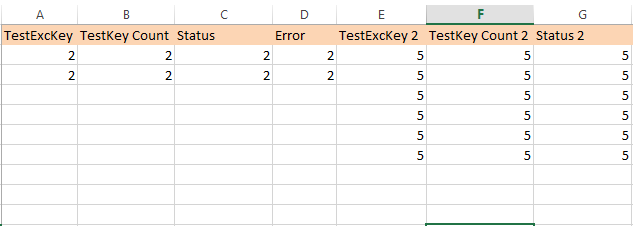# How to count rows count saperately in one table

Helo,

i need to read count rows of of A- D and E - G saperately.
How can iachieve thatrequired first count 2
second count as 6

@Mathkar_kunal

you can try to separate into different datatable then filter on empty rows and get the count

`dt.DefaultView.ToTable(False,"Col1","Col2")` - This gives datatable with only mentioned columns

once you get it use `dtrequired.Where(function(x) x.ItemArray.All(function(y) String.IsNullOrEmpty(y.Trim))).Count` to get the required count

cheers

**Finaldt = dt.DefaultView.ToTable(False,”yourcolumnname”,“Yourcolumname”)

use Int_rowcount = Finaldt.Rows.Count

1 Like

i need count sapertly for both

Use Invoke VBA activity with below code based on your required column.

Private Function Range_End_Method (sht)

Sheets(sht).Activate

Dim lRow1 As Long
Dim lRow2 As Long
Dim LastRowIdx As Long

``````lRow1 = Cells(Rows.Count, 1).End(xlUp).Row

lRow2 = Cells(Rows.Count, 2).End(xlUp).Row

If lRow1 = lRow2 Then
LastRowIdx = lRow1
Else
LastRowIdx = WorksheetFunction.Max(lRow1, lRow2)
End If
``````

Range_End_Method=LastRowIdx

End Function

AtoD_Dt= dt.DefaultView.ToTable(False,"TestExcKey”,“TestKey Count”,“Status”,“Error”)
rowCount=AtoD_Dt.rows.count

EtoG_Dt= dt.DefaultView.ToTable(False,"TestExcKey2”,“TestKey Count2”,“status 2”)
rowCount=EtoG_Dt.rows.count

Hello @Mathkar_kunal,
Try this

1. Read the Excel file and store in Variable like DT
2. Filter the data table with Output Columns with Keep condition and mention the Column index or Column name. and store in variable like DT_Filter
3. Then Filter the Rows with remove where 4 columns are empty. NAd can save in same variable
4. Use the below syntax to get the row count
``````DT_Filter.Rows.Count.Tostring
``````

DT.Dataview.ToTable(False,“TestExckey”,“Testkey Count”,“Status”,“Error”)
Count1=DT.rows.count
DT1.Dataview.ToTable(False,“TestExckey 2”,“Testkey Count2”,“Status2”)
Count2=DT1.rows.count

use this

Hey @Mathkar_kunal ,

Try out this linq

``````
(From col In dt.Columns
Let val=(From row In dt
Where Not String.IsNullOrEmpty(row(col.ToString).ToString)).ToList
Select val.Count).ToList
``````

Replace the dt with your dt variable

Hope it helps You